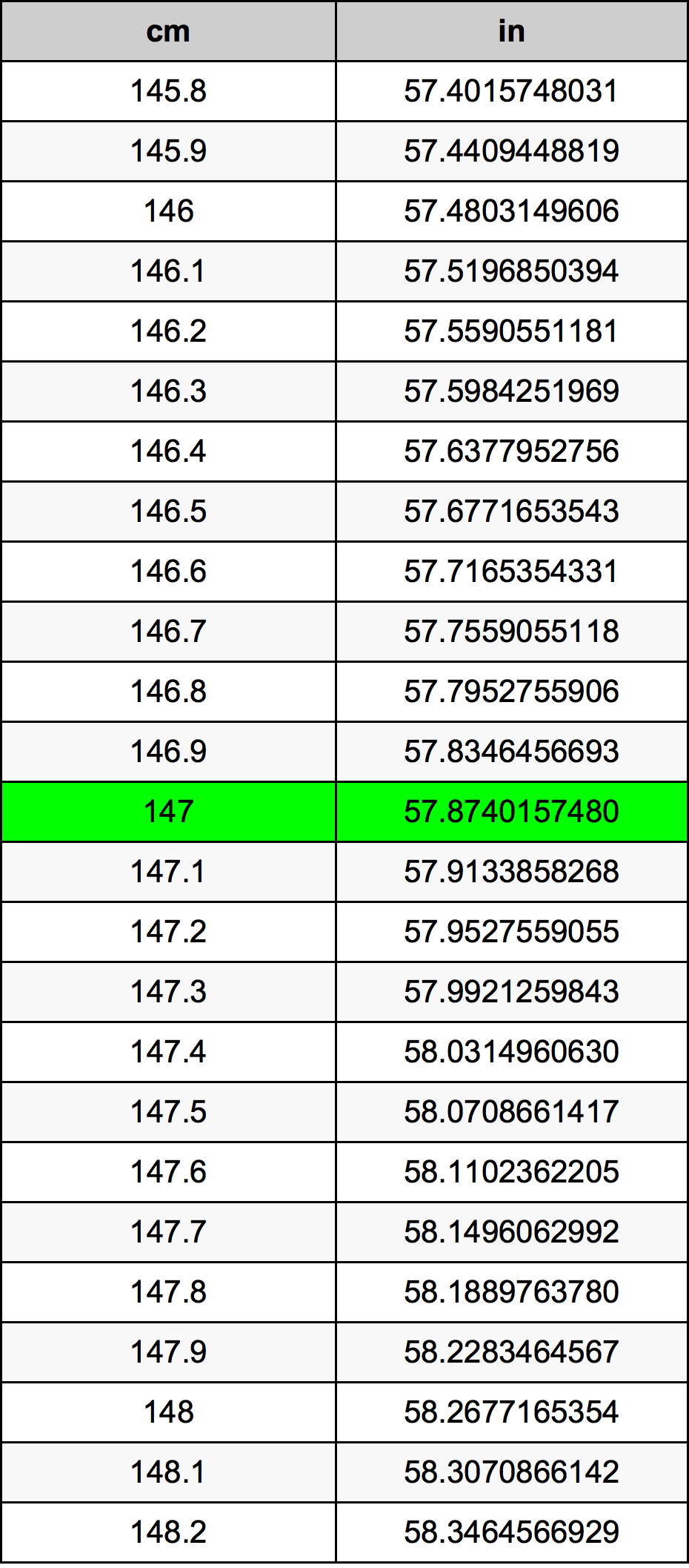Cm To Inches

# 147 cm to in147 Centimeters to Inches

cm
=
in

## How to convert 147 centimeters to inches?

 147 cm * 0.3937007874 in = 57.874015748 in 1 cm
A common question is How many centimeter in 147 inch? And the answer is 373.38 cm in 147 in. Likewise the question how many inch in 147 centimeter has the answer of 57.874015748 in in 147 cm.

## How much are 147 centimeters in inches?

147 centimeters equal 57.874015748 inches (147cm = 57.874015748in). Converting 147 cm to in is easy. Simply use our calculator above, or apply the formula to change the length 147 cm to in.

## Convert 147 cm to common lengths

UnitLength
Nanometer1470000000.0 nm
Micrometer1470000.0 µm
Millimeter1470.0 mm
Centimeter147.0 cm
Inch57.874015748 in
Foot4.8228346457 ft
Yard1.6076115486 yd
Meter1.47 m
Kilometer0.00147 km
Mile0.0009134157 mi
Nautical mile0.0007937365 nmi

## What is 147 centimeters in in?

To convert 147 cm to in multiply the length in centimeters by 0.3937007874. The 147 cm in in formula is [in] = 147 * 0.3937007874. Thus, for 147 centimeters in inch we get 57.874015748 in.

## 147 Centimeter Conversion Table## Alternative spelling

147 cm to Inches, 147 cm in Inches, 147 Centimeters to Inches, 147 Centimeters in Inches, 147 Centimeter to in, 147 Centimeter in in, 147 Centimeters to in, 147 Centimeters in in, 147 cm to Inch, 147 cm in Inch, 147 Centimeters to Inch, 147 Centimeters in Inch, 147 cm to in, 147 cm in in